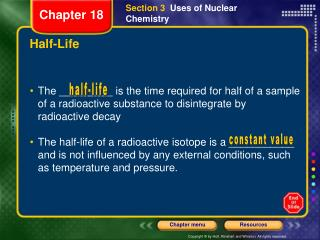DownloadDownload PresentationHalf-Life

# Half-Life

Télécharger la présentation## Half-Life

- - - - - - - - - - - - - - - - - - - - - - - - - - - E N D - - - - - - - - - - - - - - - - - - - - - - - - - - -
##### Presentation Transcript

1. Section3 Uses of Nuclear Chemistry Chapter 18 Half-Life • The _________ is the time required for half of a sample of a radioactive substance to disintegrate by radioactive decay • The half-life of a radioactive isotope is a ___________ and is not influenced by any external conditions, such as temperature and pressure. half-life constant value

2. Section3 Uses of Nuclear Chemistry Chapter 18 Half-Life • Radioisotope Half-lifeRadioisotopeHalf-life • Bismuth-212 60.5 min Polonium-215 0.0018 sec • Carbon-14 5930 yrs Polonium-216 0.16 sec • Chlorine-36 400 000 yrs Radium-226 1602 yrs • Cobalt-60 5.26 yrs Sodium-24 15.0 hrs • Iodine-131 8.14 days Strontium-90 19.9 yrs • Iron-59 46.3 days Uranium-235 710 mill yrs • Phosphorus-32 14.3 days Uranium-238 4.5 bill yrs

3. Section3 Uses of Nuclear Chemistry Chapter 18 Half-Life • Example Problem: • 212 Bi 0 b+ 212 Po • 83 - 1 84 • If you start with 200 g, how much is left after 181.5 min? • Answer: • The half-life of bismuth-212 is ___________. • How much is left after one half-life (60.5 minutes)? • How much is left after 2 half-lives (121 minutes)? • How much is left after 3 half-lives (181.5 minutes)? 60.5 minutes 100 g 50 g 25 g

4. Section3 Uses of Nuclear Chemistry Chapter 18 Half-Life • Practice Problem: • Radium-226 undergoes alpha decay. • Write the transmutation equation for this change. • What is the name if the new substance that is produced? • If you start with 4000 g, how much will be left after 6408 years? • Answers: • (A) • (B) Radon-222 226Ra 88 4a + 2 222Rn 86

5. Section3 Uses of Nuclear Chemistry Chapter 18 Half-Life • Practice Problem (continued): • Radium-226 undergoes alpha decay. (C) If you start with 4000 g, how much will be left after 6408 years? Answer: What is the half-life of Radium-226? How much is left after 1 half-life? How much is left after 2 half-lives (3204 years)? How much is left after 3 half-lives (4806 years)? How much is left after 4 half-lives (6408 years)? 1602 years 2000 g 1000 g 500 g 250 g

6. Section3 Uses of Nuclear Chemistry Chapter 18 Half-Life • Practice Problem: • Carbon-14 undergoes beta decay. • Write the transmutation equation for this change. • What is the name if the new substance that is produced? • If you start with 600 g, how much will be left after 17,790 years? • Answers: 14C 6 0b + -1 14N 7 (A) (B) Nitrogen-14

7. Section3 Uses of Nuclear Chemistry Chapter 18 Half-Life • Practice Problem (continued): • Carbon-14 undergoes beta decay. • (C) If you start with 600 g, how much will be left after 17,790 years? • Answer: • What is the half-life of carbon-14? • How much is left after 1 half-life? • How much is left after 2 half-lives (11,860 years)? • How much is left after 3 half-lives (17,790 years)? 5930 years 300 g 150 g 75 g